# Algebra 1 : How to find the percent of increase

## Example Questions

### Example Question #51 : How To Find The Percent Of Increase

Find the percent of increase from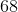to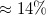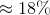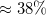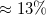Explanation:

For this type of problem we use this formula: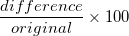.

"Difference" is simply the difference between the two numbers given, and "original" is the number that is stated usually after the word "from" , example: from____ to ____.

In this problem our formula will be filled in as follows: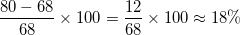.

This is our percent increase.

### Example Question #52 : How To Find The Percent Of Increase

Find the percent of increase fromto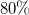Explanation:

For this type of problem we use this formula:.

"Difference" is simply the difference between the two numbers given, and "original" is the number that is stated usually after the word "from" , example: from____ to ____.

In this problem our formula will be filled in as follows: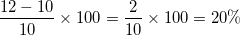.

This is our percent increase.

### Example Question #53 : Percent Of Change

Find the percent increase fromto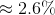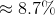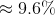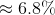Explanation:

For this type of problem we use this formula:. "Difference" is simply the difference between the two numbers given, and "original" is the number that is stated usually after the word "from" , example: from ____ to ____. In this problem our formula will be filled in as follows: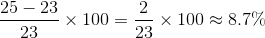. This is our percent increase.

### Example Question #51 : Percent Of Change

Find the percent increase from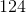to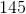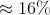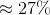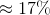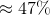Explanation:

For this type of problem we use this formula:. "Difference" is simply the difference between the two numbers given, and "original" is the number that is stated usually after the word "from" , example: from ____ to ____. In this problem our formula will be filled in as follows: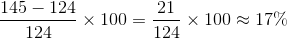. This is our percent increase.

### Example Question #52 : Percent Of Change

Find the percent increase fromto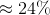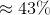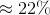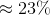Explanation:

For this type of problem we use this formula:. "Difference" is simply the difference between the two numbers given, and "original" is the number that is stated usually after the word "from" , example: from ____ to ____. In this problem our formula will be filled in as follows: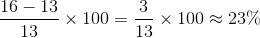. This is our percent increase.

### Example Question #53 : Percent Of Change

Find the percent increase fromto.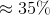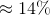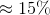Explanation:

For this type of problem we use this formula:. "Difference" is simply the difference between the two numbers given, and "original" is the number that is stated usually after the word "from" , example: from ____ to ____. In this problem our formula will be filled in as follows: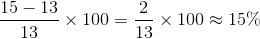. This is our percent increase.

### Example Question #54 : Percent Of Change

Find the percent increase fromto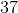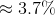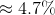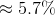Explanation:

For this type of problem we use this formula:. "Difference" is simply the difference between the two numbers given, and "original" is the number that is stated usually after the word "from" , example: from ____ to ____. In this problem our formula will be filled in as follows: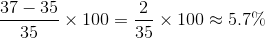. This is our percent increase.

### Example Question #55 : Percent Of Change

Find the percent increase fromto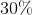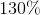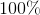Explanation:

For this type of problem we use this formula:. "Difference" is simply the difference between the two numbers given, and "original" is the number that is stated usually after the word "from" , example: from ____ to ____. In this problem our formula will be filled in as follows: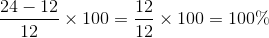. This is our percent increase.

### Example Question #51 : Percent Of Change

Find the percent increase fromto.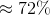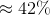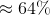Explanation:

For this type of problem we use this formula:. "Difference" is simply the difference between the two numbers given, and "original" is the number that is stated usually after the word "from" , example: from ____ to ____. In this problem our formula will be filled in as follows: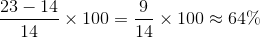. This is our percent increase.

### Example Question #52 : Percent Of Change

Find the percent increase fromto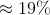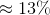For this type of problem we use this formula:. "Difference" is simply the difference between the two numbers given, and "original" is the number that is stated usually after the word "from" , example: from ____ to ____. In this problem our formula will be filled in as follows: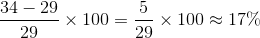. This is our percent increase.